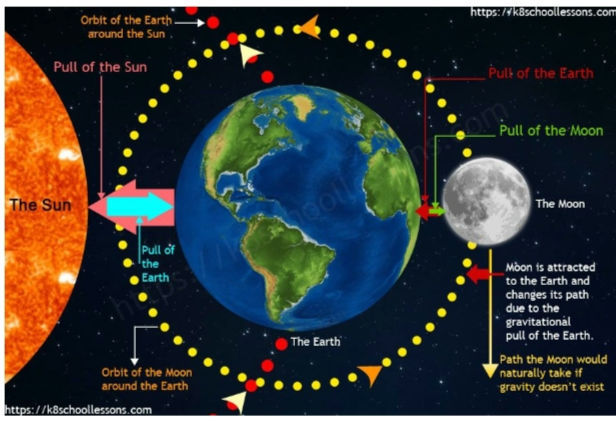top of page
Search
• astroteens259

# How does Gravity Affect Time?

~ Deepthy

In order to understand how time is affected by gravity, we need to first realise what time is. Defined by its measurement, time is a continuous sequence of existence. Denoted by t, time is a relative quantity that depends on the frame of reference. It is conventionally measured in terms of seconds, minutes, hours, days etc.Now that we know how time is defined, we need to understand what gravity is and what it does, to comprehend the relation between the two.  The weakest of the four fundamental forces in nature, gravity is the universal force of attraction between all things with mass or energy.  Gravity is measured by the acceleration (denoted by g) that it gives to freely falling objects. g is about 9.8 metres per second squared at the earth’s surface.Source: The Guardian

Newton’s law of universal gravitation states that every particle attracts every other particle in the universe with a force that is directly proportional to the product of their masses and inversely proportional to the square of the distance between their centres.where F is the force, m1 and m2 are the masses of the objects, r is the distance between the centres of the masses and G is the gravitational constant, equal to 6.67408 × 10-11 m3 kg-1 s-2Source: The Guardian Einstein’s theory of General Relativity refined Newton’s Law of Universal Gravitation. Einstein suggested that gravity is the dynamical curvature of the universe, which is caused by heavy bodies and determines the path that objects travel.  To put it simply, gravity is a consequence of space-time curvature as the flow of time is not constant throughout the universe but varies according to the distribution of massive bodies. The force of gravity is stronger towards the surface of Earth, as the curvature is more intense there than on the fringes.Source: Wikipedia Let’s now look at how gravity actually affects time!  According to Einstein’s theory of General Relativity, the fabric of space-time is stretched and contracted by matter such that objects are pushed downwards, with the curvature of space-time accelerating objects that move downward.  Einstein proposed that massive objects distort the universe by curving or warping it, resulting in other objects moving on or along those curves—something we experience as gravity. Its speed not only equals that of light precisely but these two constants must be exactly equal or General Relativity would fall apart.It is so significant an effect that it requires that the Global Positioning System's artificial satellites have their clocks corrected as the clocks on these satellites tick faster than the clocks on Earth’s surface. So corrections are made to ensure that the GPS data sent back to Earth’s surface have matching times. To conclude, gravity is the curvature of space-time. It slows down the flow of time i.e the heavier the object, the stronger its gravitational field and hence the slower time passes in its vicinity. Referred sources: https://byjus.com/physics/the-idea-of-time/ https://en.wikipedia.org/wiki/Time https://en.wikipedia.org/wiki/Time https://en.wikipedia.org/wiki/Gravity https://www.britannica.com/science/gravity-physics https://physicsworld.com/a/gravitys-effect-on-time-confirmed/ https://www.forbes.com/sites/startswithabang/2019/10/24/this-is-why-the-speed-of-gravity-must-equal-the-speed-of-light/?sh=265604862fc0 https://www.science.org.au/curious/space-time/gravity https://www.wtamu.edu/~cbaird/sq/2013/06/24/does-time-go-faster-at-the-top-of-a-building-compared-to-the-bottom/#:~:text=Gravitational%20time%20dilation%20occurs%20because,the%20slower%20time%20itself%20proceeds. https://en.wikipedia.org/wiki/Gravitational_redshift